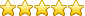Quick Help

Knowledgebase is a categorized collection of answers to frequently asked questions (FAQ) and articles. You can read articles in this category or select a subcategory that you are interested in.

# Question

When I watch a parsed value in the main window I see a parser item name, value and a number in square brackets. E.g.  or . What is it?

Example:

DATE_TIME_STAMP=2017-02-27 08:54:34;DATA_SOURCE_FULL_NAME="192.168.0.132:50000"

The number in brackets means a data type of a parsed value:

2 - Smallint (signed integer, 16 bit, S16).
3 - Integer (signed integer, 32 bit, S32).
4 - Single (float value, single precision, 32 bit, F32).
5 - Double (float value, double precision, 64 bit, F32).
6 - Currency (float value, double precision, 64 bit, F64).
7 - Date, Time or Timestamp (encoded as YYYY-MM-DD HH:NN:SS, 24H format).
8 - String (variable length of ASCII characters).
14 - Decimal (signed integer, 32 bit, S32).
16 - ShortInt (signed integer, 8 bit, S8).
17 - Byte (unsigned integer, 8 bit, U8).
18 - Word (unsigned integer, 16 bit, U16).
19 - DWord (unsigned integer, 32 bit, U32).
20 - Int64 (signed integer, 64 bit, S64).
21 - Unsigned Int64 (unsigned integer, 64 bit, U64).

Rating (Votes):(1)